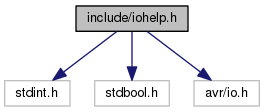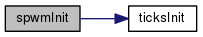ATtiny85 Template Code Code snippets for the ATtiny85
iohelp.h File Reference
`#include <stdint.h>`
`#include <stdbool.h>`
`#include <avr/io.h>`
Include dependency graph for iohelp.h:This graph shows which files directly or indirectly include this file:Go to the source code of this file.

## Macros

#define pinHigh(pin)   PORTB |= (1 << pin)

#define pinLow(pin)   PORTB &= ~(1 << pin)

#define pinGet(pin)   (PINB & (1 << pin))

## Typedefs

typedef enum _ANALOG ANALOG

typedef enum _PWM PWM

typedef enum _SPWM SPWM

## Enumerations

enum  _ANALOG {
}

enum  _PWM { PWM0 = 0, PWM1 = 1 }

enum  _SPWM { SPWM0 = 0, SPWM1 = 1, SPWM2 = 2, SPWM3 = 3 }

## Functions

void pinSet (uint8_t pin, bool value)

void pwmInit ()

void pwmOut (PWM pwm, uint8_t value)

void spwmInit ()

void spwmOut (SPWM pwm, uint8_t value)

uint8_t spwmValue (SPWM pwm)

void sspiInit (uint8_t sck, uint8_t mosi, uint8_t miso)

void sspiOutMSB (uint8_t sck, uint8_t mosi, uint16_t data, uint8_t bits)

uint16_t sspiInMSB (uint8_t sck, uint8_t miso, uint8_t bits)

uint16_t sspiInOutMSB (uint8_t sck, uint8_t mosi, uint8_t miso, uint16_t data, uint8_t bits)

void sspiOutLSB (uint8_t sck, uint8_t mosi, uint16_t data, uint8_t bits)

uint16_t sspiInLSB (uint8_t sck, uint8_t miso, uint8_t bits)

uint16_t sspiInOutLSB (uint8_t sck, uint8_t mosi, uint8_t miso, uint16_t data, uint8_t bits)

## Macro Definition Documentation

 #define pinGet ( pin ) (PINB & (1 << pin))

Get the state of a pin

Parameters
 pin the pin number to read

Definition at line 55 of file iohelp.h.

 #define pinHigh ( pin ) PORTB |= (1 << pin)

Macro to set a pin high

Set the output value of a pin on port B high

Parameters
 pin the pin number to change

Definition at line 30 of file iohelp.h.

 #define pinLow ( pin ) PORTB &= ~(1 << pin)

Set a pin low

Set the output value of a pin on port B low

Parameters
 pin the pin number to change

Definition at line 39 of file iohelp.h.

## Typedef Documentation

 typedef enum _ANALOG ANALOG

Analog pin inputs

 typedef enum _PWM PWM

PWM outputs

This enumerates the available PWM outputs.

 typedef enum _SPWM SPWM

Software PWM outputs

This enumerates the available outputs for software generated PWM.

## Enumeration Type Documentation

 enum _ANALOG

Analog pin inputs

Enumerator

Definition at line 64 of file iohelp.h.

 enum _PWM

PWM outputs

This enumerates the available PWM outputs.

Enumerator
PWM0

PWM output 0 (PB0)

PWM1

PWM output 1 (PB1)

Definition at line 104 of file iohelp.h.

 enum _SPWM

Software PWM outputs

This enumerates the available outputs for software generated PWM.

Enumerator
SPWM0

Software PWM output 0 (defined by SPWM_PIN0)

SPWM1

Software PWM output 1 (defined by SPWM_PIN1)

SPWM2

Software PWM output 2 (defined by SPWM_PIN2)

SPWM3

Software PWM output 3 (defined by SPWM_PIN3)

Definition at line 134 of file iohelp.h.

## Function Documentation

Initialise the specified analog pin

This function initialises the ADC accessory as well as setting up the input pin attached to the ADC. The set up uses VCC as the reference voltage. If you need to change this do so after initialising all the ADC inputs you want to use.

Parameters

Definition at line 20 of file analog.c.

Read a 10 bit value from the specified input with option input skipping and averaging.

Parameters
 adc the ADC input to read skip the number of samples to skip (read and ignore). average the number of samples to average (must be >= 1)
Returns
the sample value.

Definition at line 61 of file analog.c.

 void pinSet ( uint8_t pin, bool value )

Set the state of a pin

Alternative function to pinHigh/pinLow macros.

Parameters
 pin the pin number to change value the value to set (true = high, false = low)
 void pwmInit ( )

Initialise the PWM system

Sets up PWM support. The library only supports hardware PWM on TIMER0 so you only get up to two PWM pins - PB0 and PB1.

Definition at line 17 of file pwm.c.

 void pwmOut ( PWM pwm, uint8_t value )

Set the value of a PWM pin

Set the output for a PWM pin.

Parameters
 pwm the PWM output to change value the value for the duty cycle ranging from 0 (fully off) to 255 (fully on).

Definition at line 30 of file pwm.c.

 void spwmInit ( )

Initialise the software PWM system

Sets up software PWM support. Using the software PWM implementation also enables the system ticks module (they share the same interrupt).

Definition at line 107 of file systicks.c.

Here is the call graph for this function:void spwmOut ( SPWM pwm, uint8_t value )

Set the value of a software PWM pin

Set the output for a software PWM pin.

Parameters
 pwm the software PWM output to change value the value for the duty cycle ranging from 0 (fully off) to 255 (fully on).

Definition at line 140 of file systicks.c.

 uint8_t spwmValue ( SPWM pwm )

Get the current value of a software PWM pin

Returns the current value of a software PWM pin.

Parameters
 pwm the software PWM output to query
Returns
the current value of the software PWM pin

Definition at line 153 of file systicks.c.

 void sspiInit ( uint8_t sck, uint8_t mosi, uint8_t miso )

Initialise pins for software SPI

It is not necessary to call this in order to use software SPI, it is simply a convenience function to do the necessary setup. You can initialise the pins directly if needed.

Parameters
 sck the pin to use for the SCK output mosi the pin to use for the MOSI output miso the pin to use for the MISO input

Definition at line 22 of file softspi.c.

 uint16_t sspiInLSB ( uint8_t sck, uint8_t miso, uint8_t bits )

Transfer data from a slave (LSB first)

Parameters
 sck the pin to use for the SCK output miso the pin to use for the MISO input bits the number of bits to transfer
Returns
a 16 bit value containing the data in the lowest bits.

Definition at line 156 of file softspi.c.

 uint16_t sspiInMSB ( uint8_t sck, uint8_t miso, uint8_t bits )

Transfer data from a slave (MSB first)

Parameters
 sck the pin to use for the SCK output miso the pin to use for the MISO input bits the number of bits to transfer
Returns
a 16 bit value containing the data in the lowest bits.

Definition at line 68 of file softspi.c.

 uint16_t sspiInOutLSB ( uint8_t sck, uint8_t mosi, uint8_t miso, uint16_t data, uint8_t bits )

Transfer data to and from a slave (LSB first)

Parameters
 sck the pin to use for the SCK output mosi the pin to use for the MOSI output miso the pin to use for the MISO input data the data to transfer out bits the number of bits to transfer
Returns
a 16 bit value containing the read data in the lowest bits.

Definition at line 184 of file softspi.c.

 uint16_t sspiInOutMSB ( uint8_t sck, uint8_t mosi, uint8_t miso, uint16_t data, uint8_t bits )

Transfer data to and from a slave (MSB first)

Parameters
 sck the pin to use for the SCK output mosi the pin to use for the MOSI output miso the pin to use for the MISO input data the data to transfer out bits the number of bits to transfer
Returns
a 16 bit value containing the read data in the lowest bits.

Definition at line 95 of file softspi.c.

 void sspiOutLSB ( uint8_t sck, uint8_t mosi, uint16_t data, uint8_t bits )

Transfer data to a slave (LSB first)

Parameters
 sck the pin to use for the SCK output mosi the pin to use for the MOSI output data the data to transfer bits the number of bits to transfer

Definition at line 129 of file softspi.c.

 void sspiOutMSB ( uint8_t sck, uint8_t mosi, uint16_t data, uint8_t bits )

Transfer data to a slave (MSB first)

Parameters
 sck the pin to use for the SCK output mosi the pin to use for the MOSI output data the data to transfer bits the number of bits to transfer

Definition at line 40 of file softspi.c.